# Arithmetical functions commutable with sums of squares

I. Kátai and B. M. Phong
Notes on Number Theory and Discrete Mathematics
Print ISSN 1310–5132, Online ISSN 2367–8275
Volume 27, 2021, Number 3, Pages 143—154
DOI: 10.7546/nntdm.2021.27.3.143-154

## Details

### Authors and affiliations

I. KátaiDepartment of Computer Algebra, University of Eötvös Loránd
1117 Budapest, Hungary

B. M. PhongDepartment of Computer Algebra, University of Eötvös Loránd
1117 Budapest, Hungary

### Abstract

Let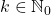and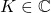, where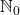,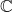denote the set of nonnegative integers and complex numbers, respectively. We give all functions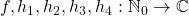which satisfy the relation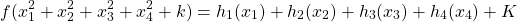for every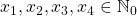. We also give all arithmetical functions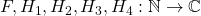which satisfy the relation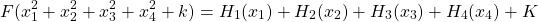for every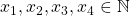, where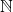denotes the set of all positive integers.

### Keywords

• Arithmetical function
• Function equation
• Sums of squares
• Lagrange’s Four-Square Theorem

• 11K65
• 11N37
• 11N64

### References

1. Bašić, B. (2014). Characterization of arithmetic functions that preserve the sum-of-squares operation. Acta Mathematica Sinica, 4, 689–695.
2. Chung, P. V. (1996). Multiplicative functions satisfying the equation f(m2+n2) = f(m2)+f(n2). Mathematica Slovaca, 46, 165–171.
3. Kátai, I., & Phong, B. M. M. (2021). A characterization of functions using Lagrange’s Four-Square Theorem. Annales Universitatis Scientiarium Budapestinensis de Rolando Eötvös Nominatae. Sectio Computatorica, 52. (accepted)
4. Kátai, I., & Phong, B. M. M. (2021). Arithmetical functions commutable with sums of squares II. Mathematica Pannonica. (submitted)
5. Khanh, B. M. M. (2017). On conjecture concerning the functional equation. Annales Universitatis Scientiarium Budapestinensis de Rolando Eötvös Nominatae. Sectio Computatorica, 46, 123–135.
6. Khanh, B. M. M. (2019). A note on a result of B. Bojan. Annales Universitatis Scientiarium Budapestinensis de Rolando Eötvös nominatae. Sectio Computatorica, 49, 285–297.
7. Khanh, B. M. M. (2021). On the equation f(n2 + Dm2 + k) = f(n)2 + Df(m)2 + k, Annales Universitatis Scientiarium Budapestinensis de Rolando Eötvös Nominatae. Sectio Computatorica, 52. (accepted)
8. Park, P.-S. (2018). Multiplicative functions commutable with sums of squares. International Journal of Number Theory, 2, 469–478.
9. Park, P.-S. (2018). On k-additive uniqueness of the set of squares for multiplicative functions. Aequationes Mathematicae, 92, 487–495.

## Cite this paper

Kátai, I., & Phong, B. M. (2021). Arithmetical functions commutable with sums of squares. Notes on Number Theory and Discrete Mathematics, 27(3), 143-154, doi: 10.7546/nntdm.2021.27.3.143-154.